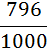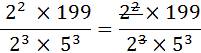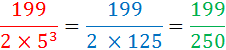# Converting Decimals To Fractions

Topic:
Fractions
Here is a worked example of how to convert a decimal number to a fraction and then simplifying it. Write 0.796 as a fraction. To do this we need to convert the .796 into a numerator and the denominator is the integer "1" followed by a number of zeroes that are equal to the number of decimal places. There are three decimal places in .796 so there are three zeroes after the number "1" (essentially what we are doing is removing the decimal point by multiplying by 1000).Simplify the fraction using Prime Factorisation 796 = 2 x 2 x 199 = 22 x 199 1000 = 2 x 2 x 2 x 5 x 5 x 5 = 23 x 53Indices in the numerator and denominator cancel each other out.